# COSMO-RS with multi-species components¶

Sometimes molecules behave in solution in ways that are not accurately captured by the “one-molecule-one-structure” paradigm of standard COSMO-RS theory. The single-structure picture of standard COSMO-RS represents much of the chemical space reasonably well but often fails to accurately describe solutions with more complex behavior. Examples of systems with complex behavior may include:

• Compounds that exist as multiple conformers
• Compounds that may dimerize, trimerize, etc.
• Compounds that can dissociate into multiple species
• Compounds or species that explicitly associate with other molecules (e.g., solvent) in solution

Multi-species COSMO-RS aims to describe the inherent complexities in these types of systems by allowing users to directly input multiple representations for a single component. The ratios of all of these species in solution are calculated and final, “apparent” values are reported for important thermodynamic values with respect to the compounds.

The following tutorial aims to guide the user in setting up and running calculations containing multi-species compounds. This guide will also discuss a few tips and point to a few GUI features that may be useful in understanding the results of the multi-species calculations. Additionally, we will walk the user through a number of practical examples demonstrating the benefits of this approach.

## Building multi-species compounds¶

The multi-species compounds input is used to organize information about a compound that can exist in multiple forms. It can be accessed in the COSMO-RS GUI with a single step:

In this window, we can input .coskf files in specific boxes which convey a relationship between a .coskf file and a single molecule of the parent compound or a molecule which must be present for an associated form to occur. .coskf files can be used directly from the AMS sigma profile database or calculated with ADF by following this tutorial. A basic guide for inputting .multiform compounds is given below:

Select Compounds → Compound with multiple forms
Input all conformers under conformers
If there are any dimers, trimers, etc., select the dropdown box next to multimers and choose the appropriate value
Enter the .coskf file and any enthalpy or entropy corrections
If the compound dissociates, place the appropriate dissociated species in the boxes under the dissociated compound section
Pay attention to the stoichiometry. Note MgCl2dissociates into 1 Mg2+and 2 Cl-ions
If any structures contain other compounds in the mixture (for association or solvation) place them in the association section.
Also, be sure to indicate which additional compounds are required in the following (1 or 2) boxes
Finally, click save to save your .multiform file

## Enthalpy and entropy corrections¶

A chemical structure’s energy in solution is calculated as the sum of two energies: (1) The bond energy, which can be seen in the Compounds → List of Added Compounds. menu; and (2) the solvent-specific corrections calculated by COSMO-RS. While this procedure generally provides acceptable relative energies for different unassociated conformers, it is usually insufficient to calculate relative energies for compounds that associate or dissociate.

It is often necessary to provide corrections for these energies. For example, in the case of acetic acid dimerization, the bond energy alone doesn’t accurately represent the enthalpy and entropy changes involved in dimerization. For this reason, we can add a correction term to the enthalpy (Hcorr) value. Of course, there is a large entropic effect associated with dimerization, but for this simple example, we’ll assume we’re performing a constant-temperature calculation and the Hcorr can effectively represent the entire correction to the free energy. Setting Hcorr to a value of 9.25 for the acetic acid dimer looks like the following: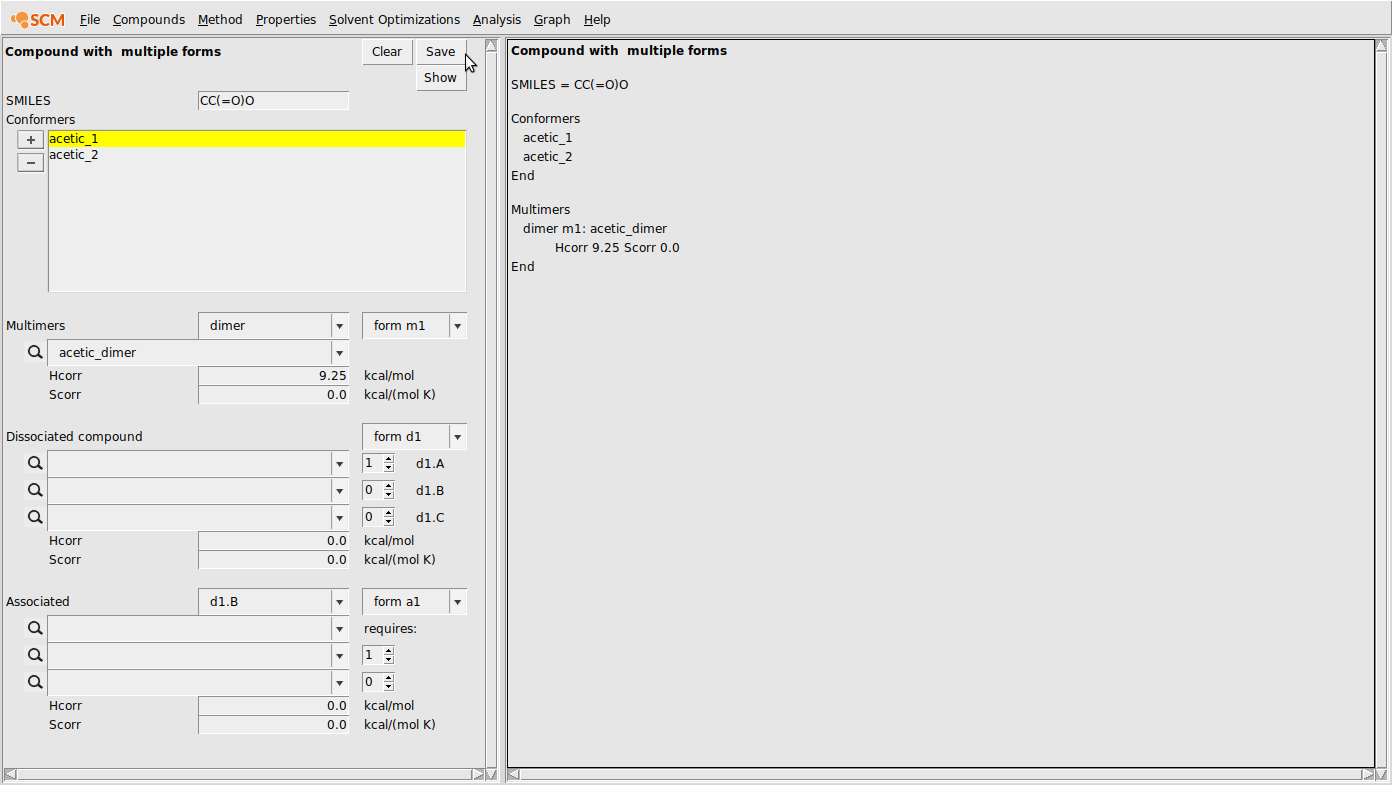## Example multi-species calculations¶

### Acetic acid dimerization¶

In this example, we’ll investigate the acetic acid/heptane and acetic acid/benzene systems. In both of these systems, acetic acid prefers to dimerize, making them good test systems to demonstrate the advantages of including multiple forms in a COSMO-RS calculation. We’ll also compare to the standard COSMO-RS approach.

First, we need to generate the .coskf files we need for this calculation. We will consider 3 geometries for acetic acid: 2 unassociated conformers and 1 dimer. These structures are shown below: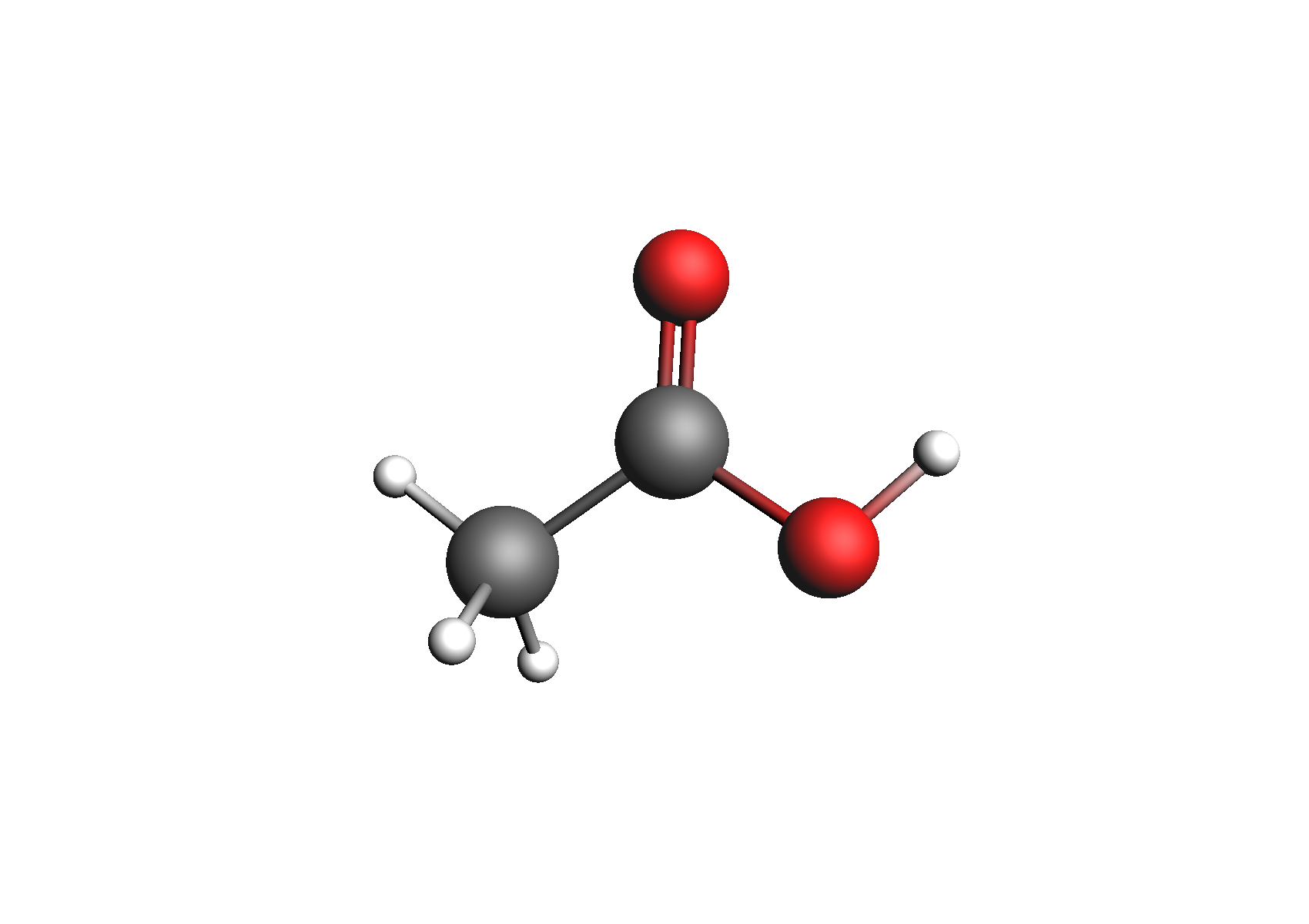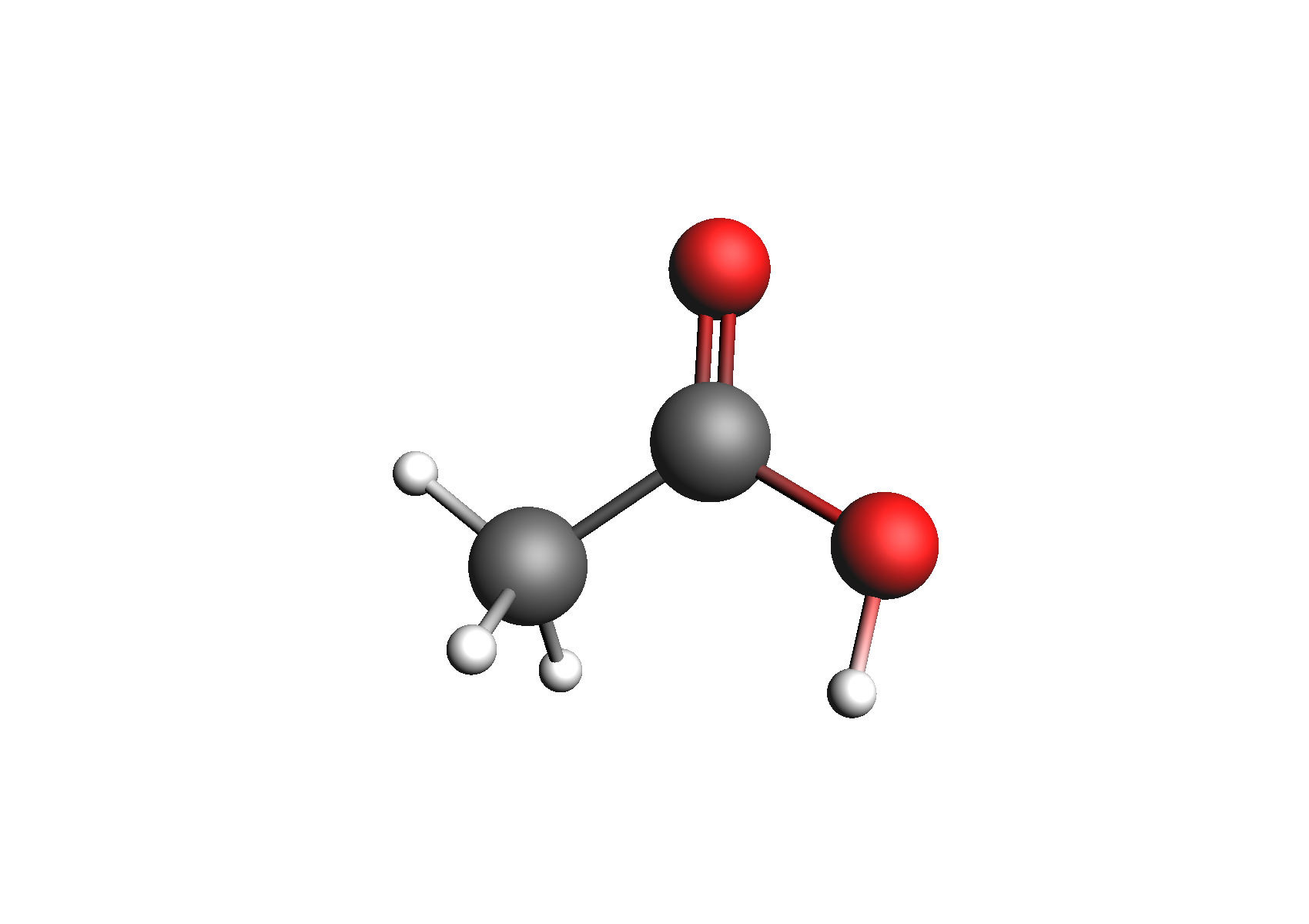Structures used to represent acetic acid: lowest energy conformer, higher energy conformer, and dimer (clockwise from top left)

Once we have these .coskf files, we must build the .multiform file for multiform acetic acid. These steps are summarized below:

Compounds → Compound with multiple forms
Add the acetic acid conformers under conformers
Add the acetic acid dimer under dimer
Set the Hcorr of the dimer to 9.25
Hit save in the top of the multiple forms box
Save as acetic_acid.multiform

Next, we’ll perform a binary mixture calculation with n-Heptane (available as Heptane.coskf in the COSMO-RS database). This can be done with the following steps:

Properties → Binary Mixture VLE/LLE
Select acetic_acid.multiform for compound 1
Select Heptane for compound 1
Set n to 20
Click Run

The result of this calculation is the following: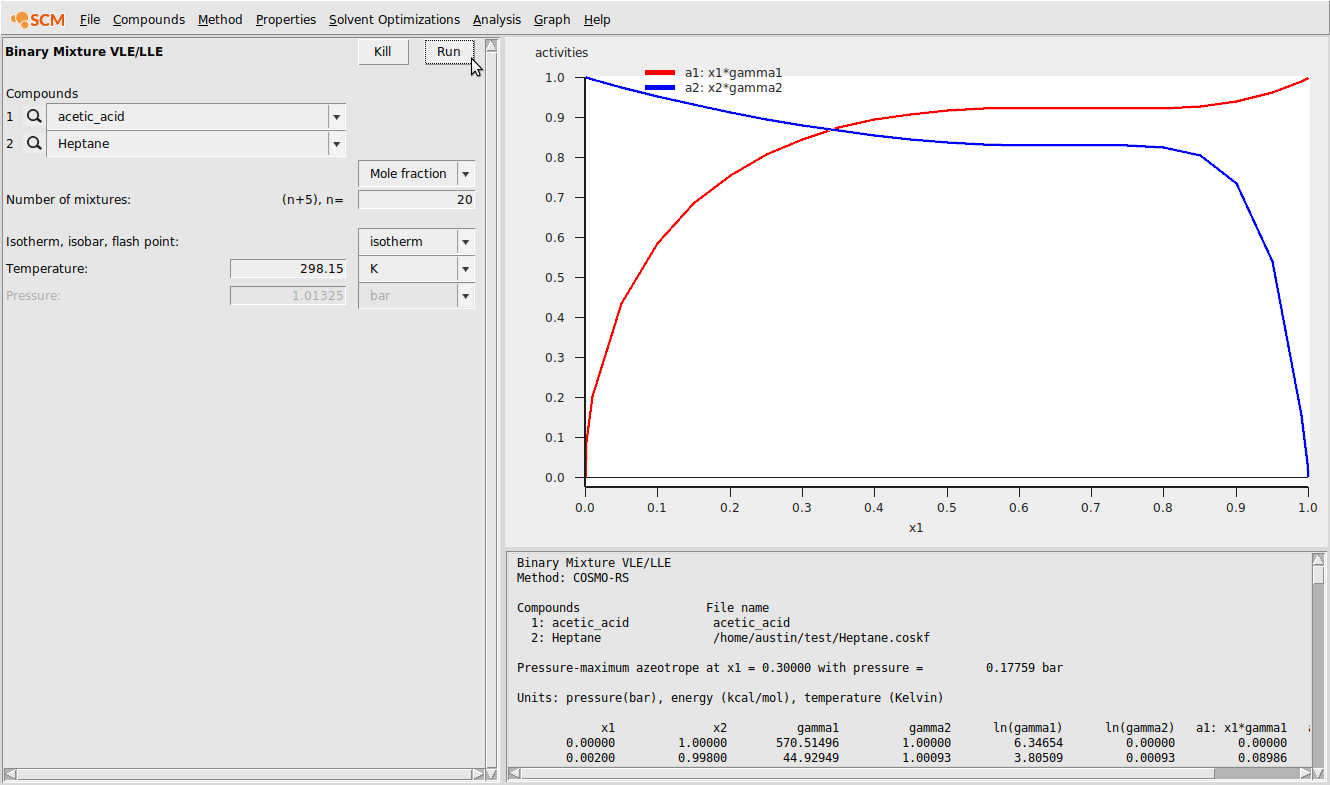We can also view the relative amounts of the different forms of acetic acid as a function of mole fraction. To view this, do the following:

Graph → Y Axis → Liquid phase composition 1

This should produce the following:Finally, we can compare the calculated activities among 3 scenarios: (1) using a single structure for acetic acid that is also not the lowest energy conformer; (2) using two conformers for acetic acid; and (3) using both conformers and the dimer.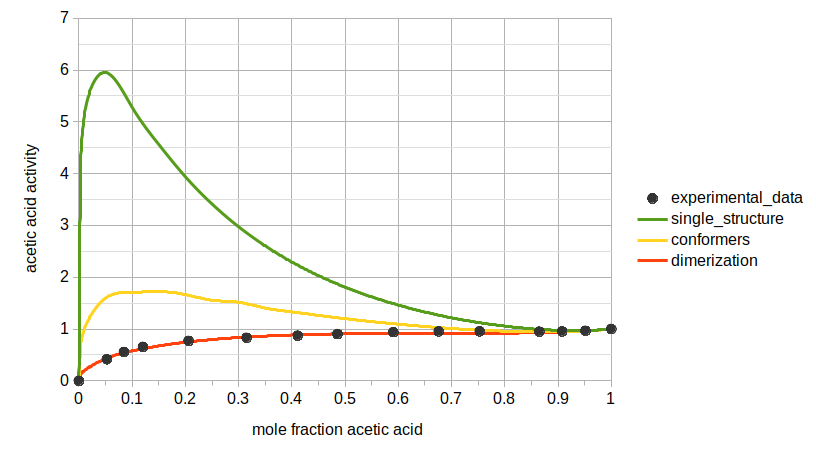### NaCl in Water¶

In this example, we will calculate mean ionic activity coefficients for a very common system: NaCl in water. Though it is possible to calculate the properties of this system with respect to NaCl as a single compound, it is more convenient for calculating mean ionic activity coefficients if we consider Na+ and Cl- as separate compounds.

One of the most important effects in this system is the solvation of the dissociated ions with water molecules. Water molecules interact strongly with the Na+ and Cl- ions in solution, and in this example we will demonstrate that including explicitly solvated species captures the behavior of the system much better than standard COSMO-RS does.

First, we need to do a series of calculations to generate .coskf files for the Na+ and Cl- ions with various degrees of hydration. The geometries of these structures are shown below: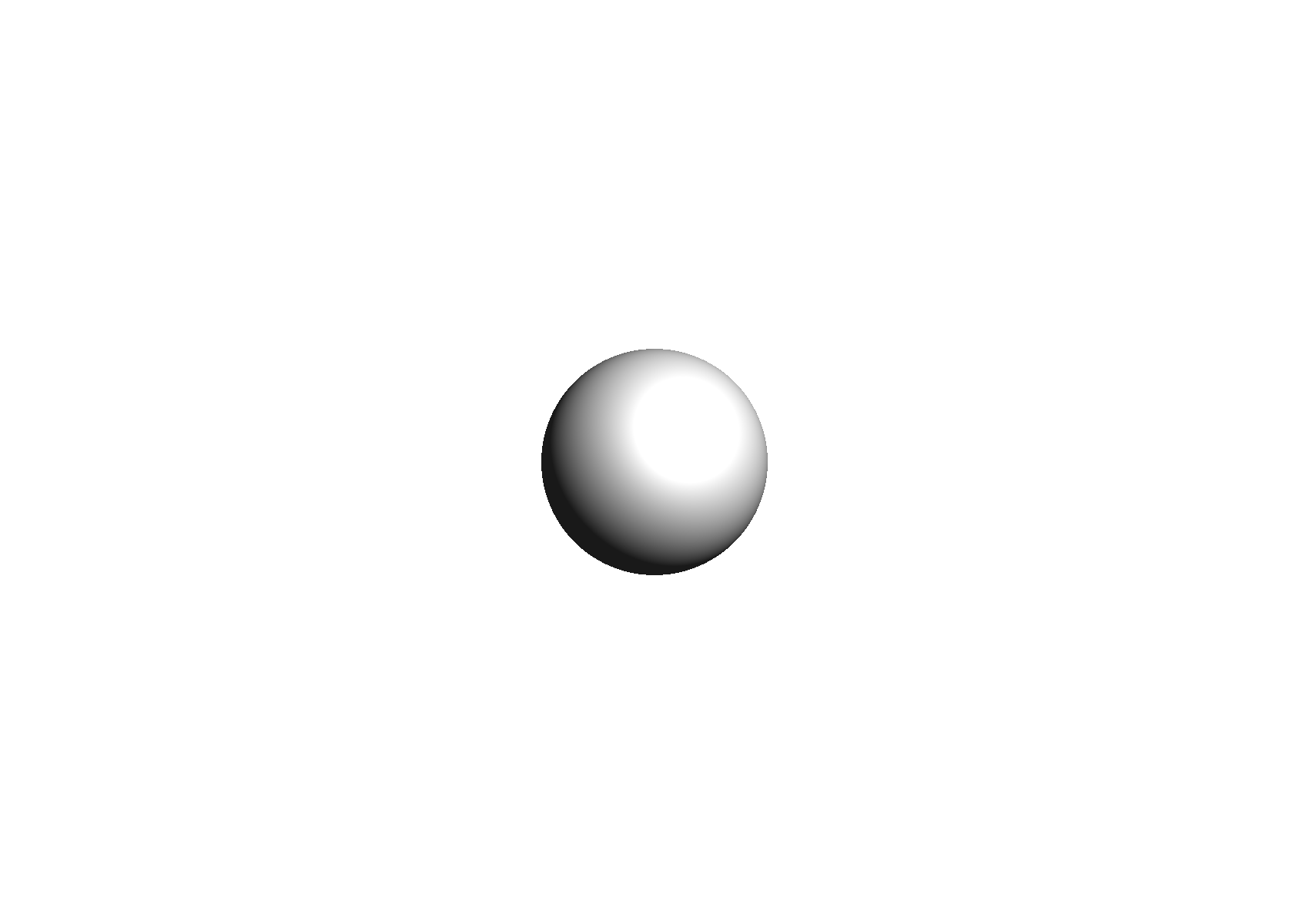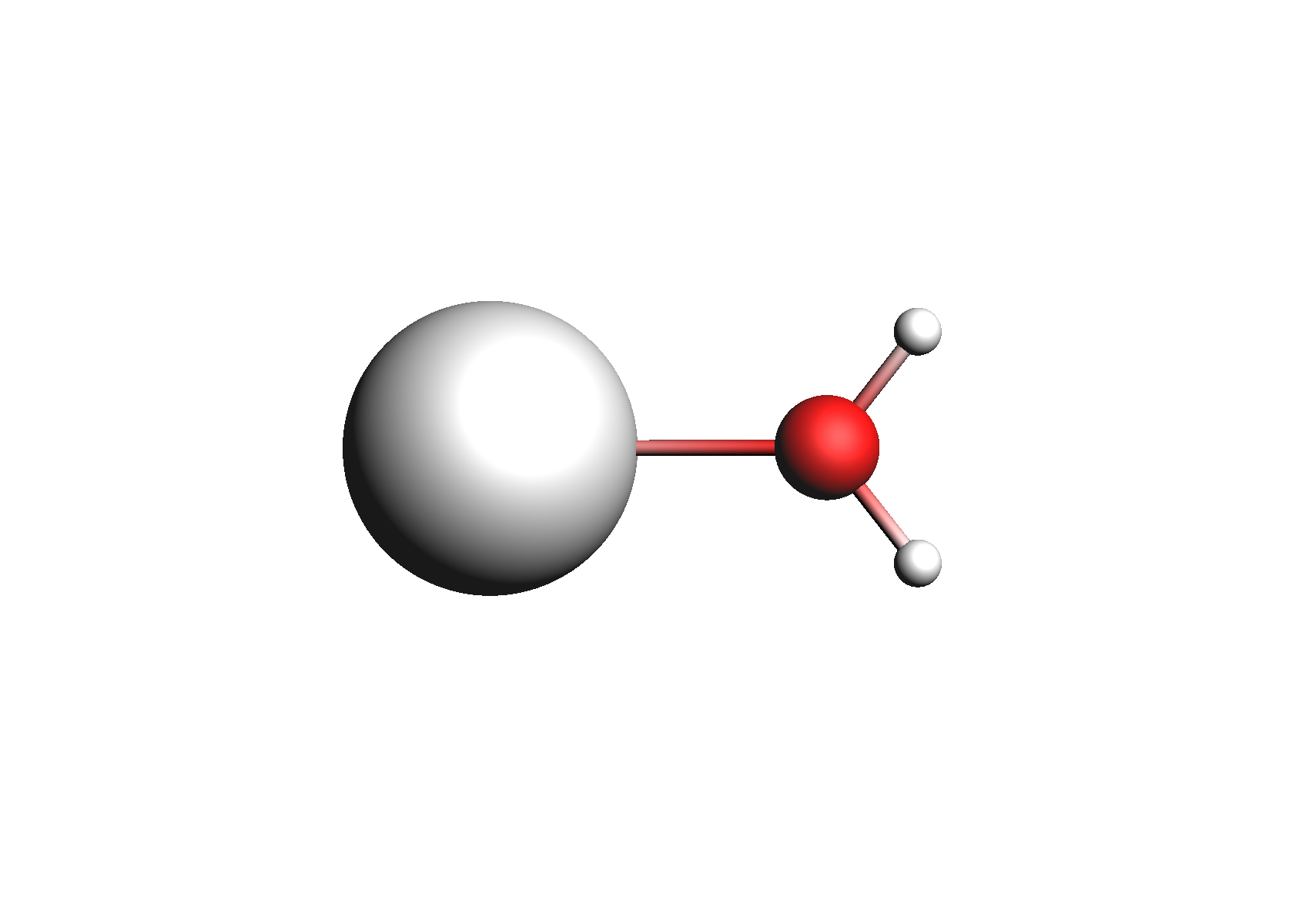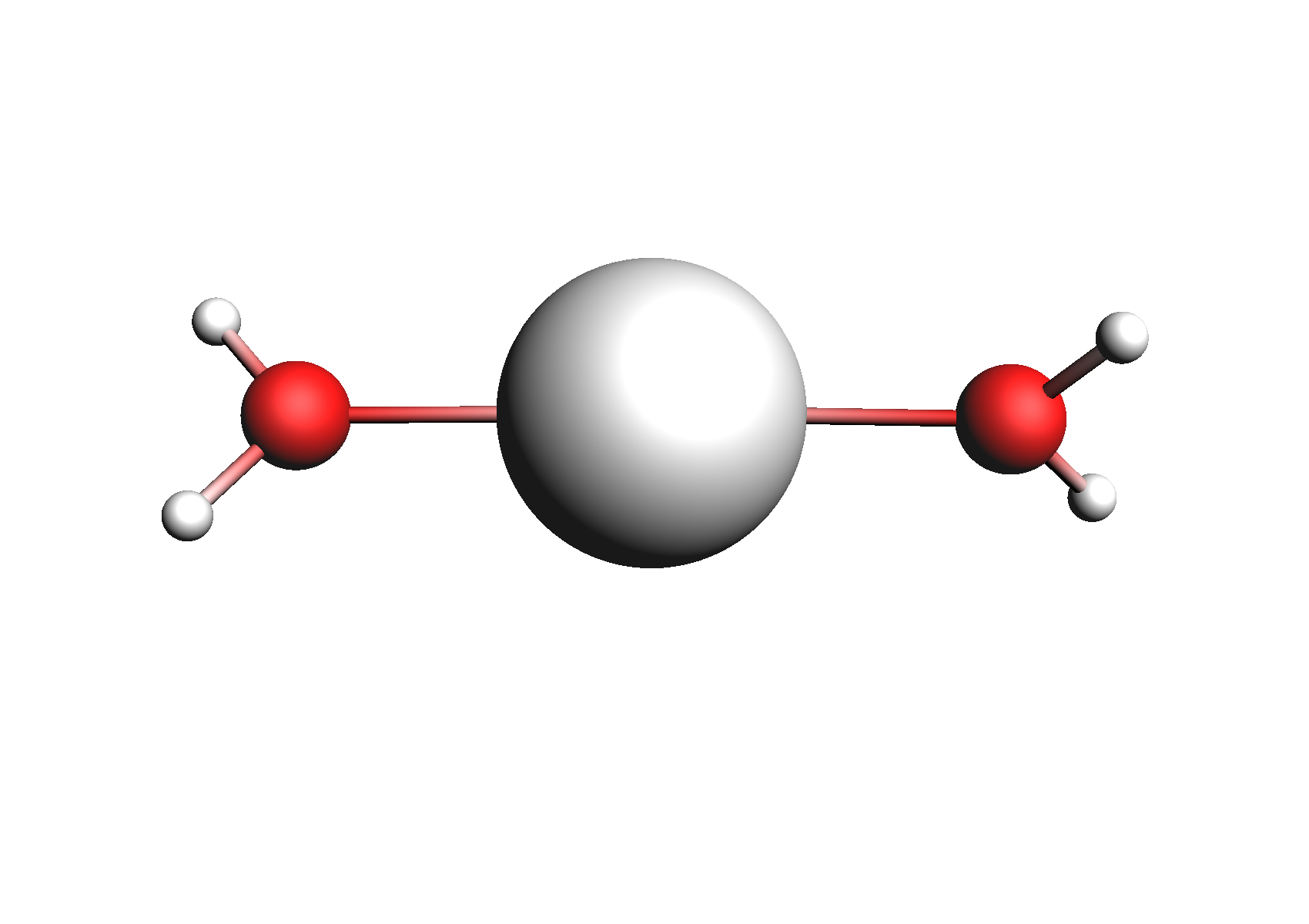Structures used for the Na+ cation (unassociated and 1,2,3,4 explicit waters)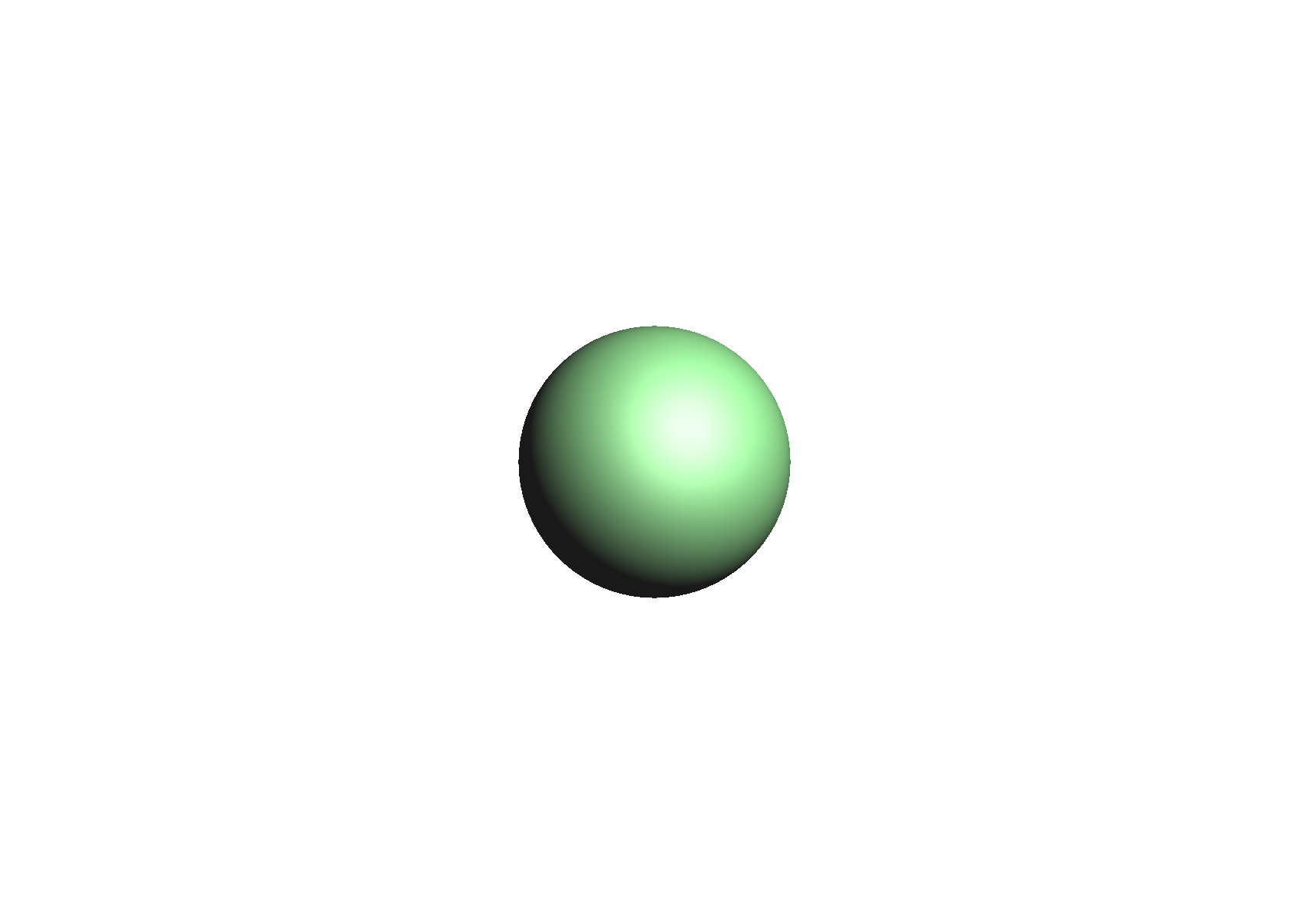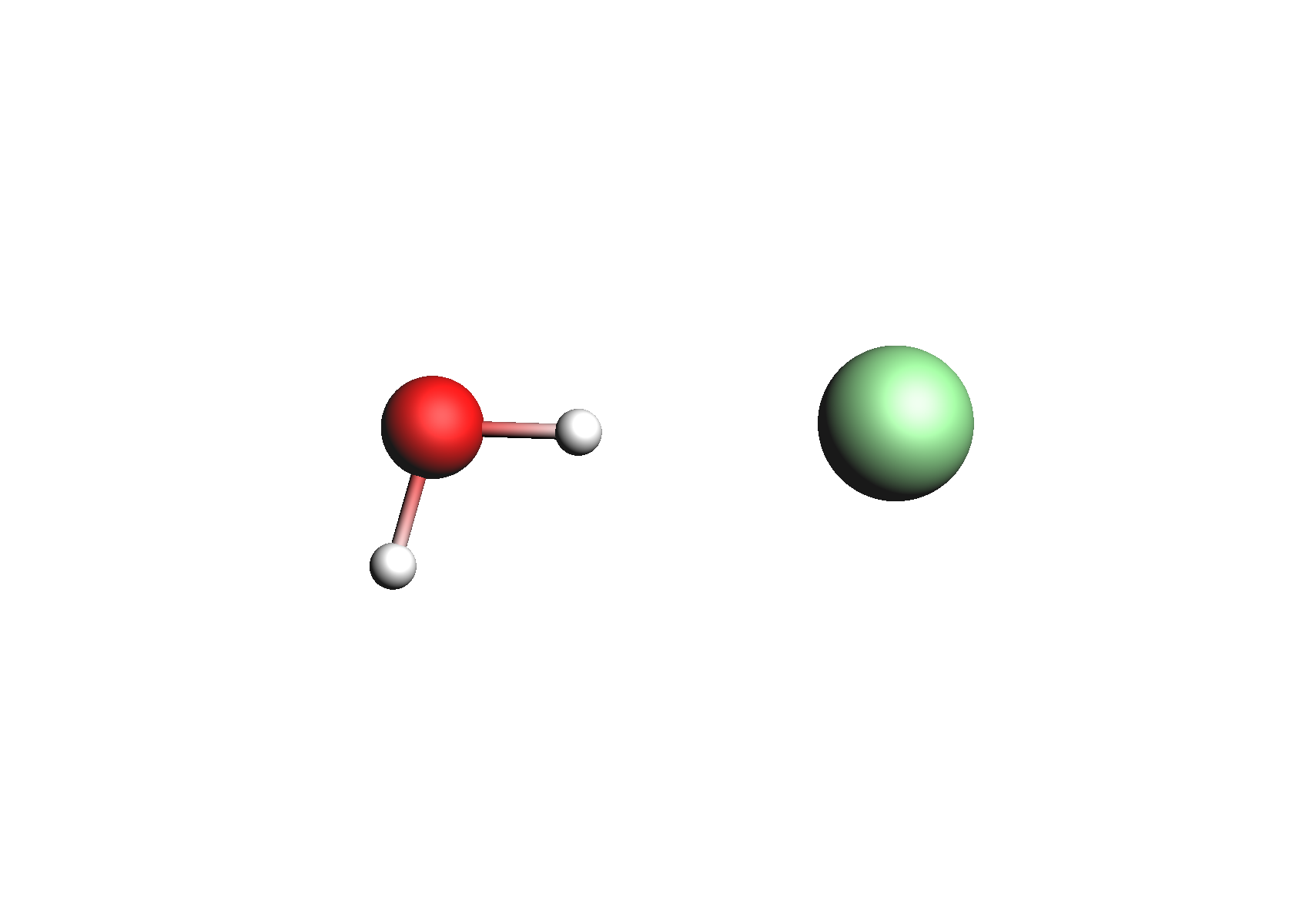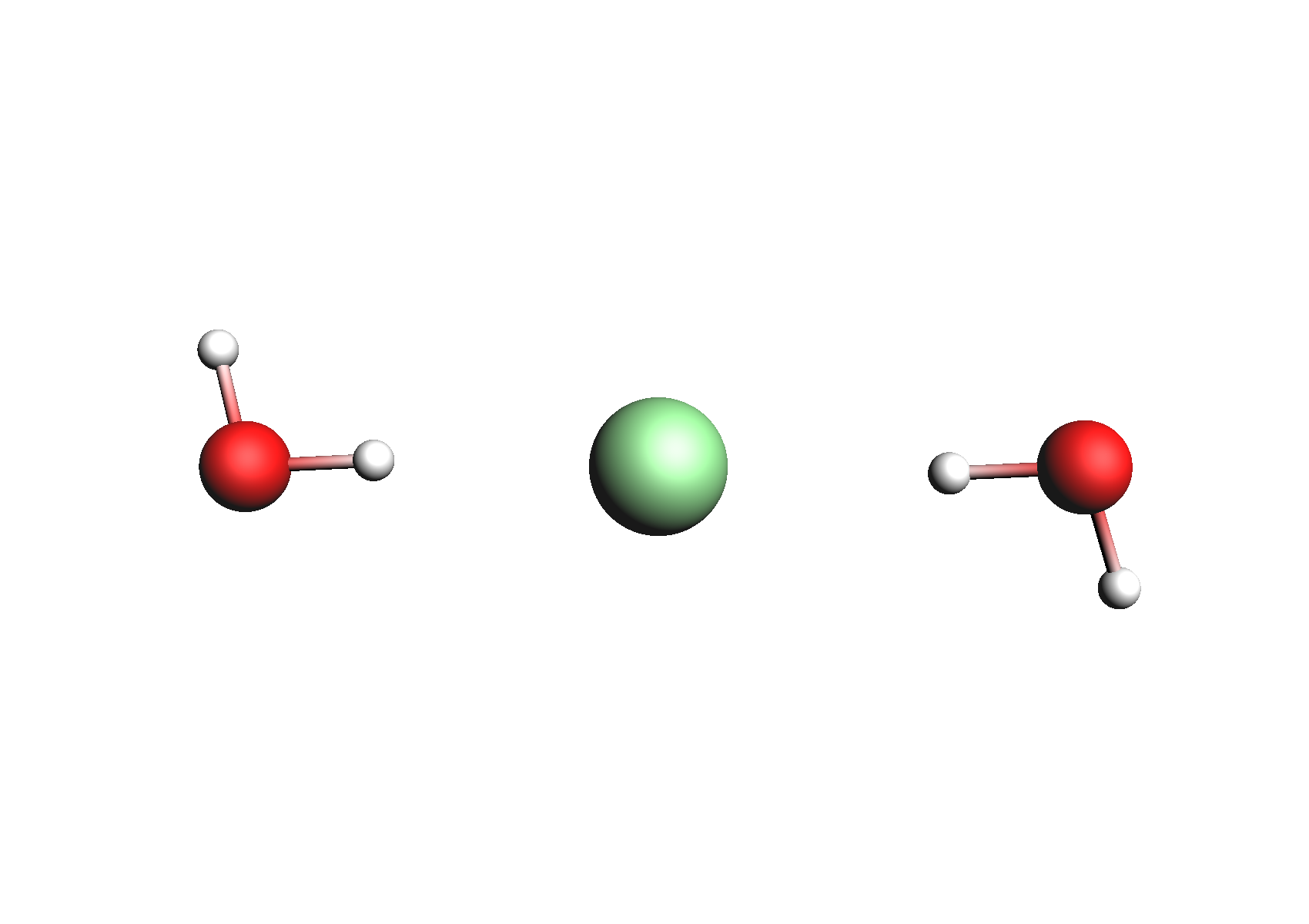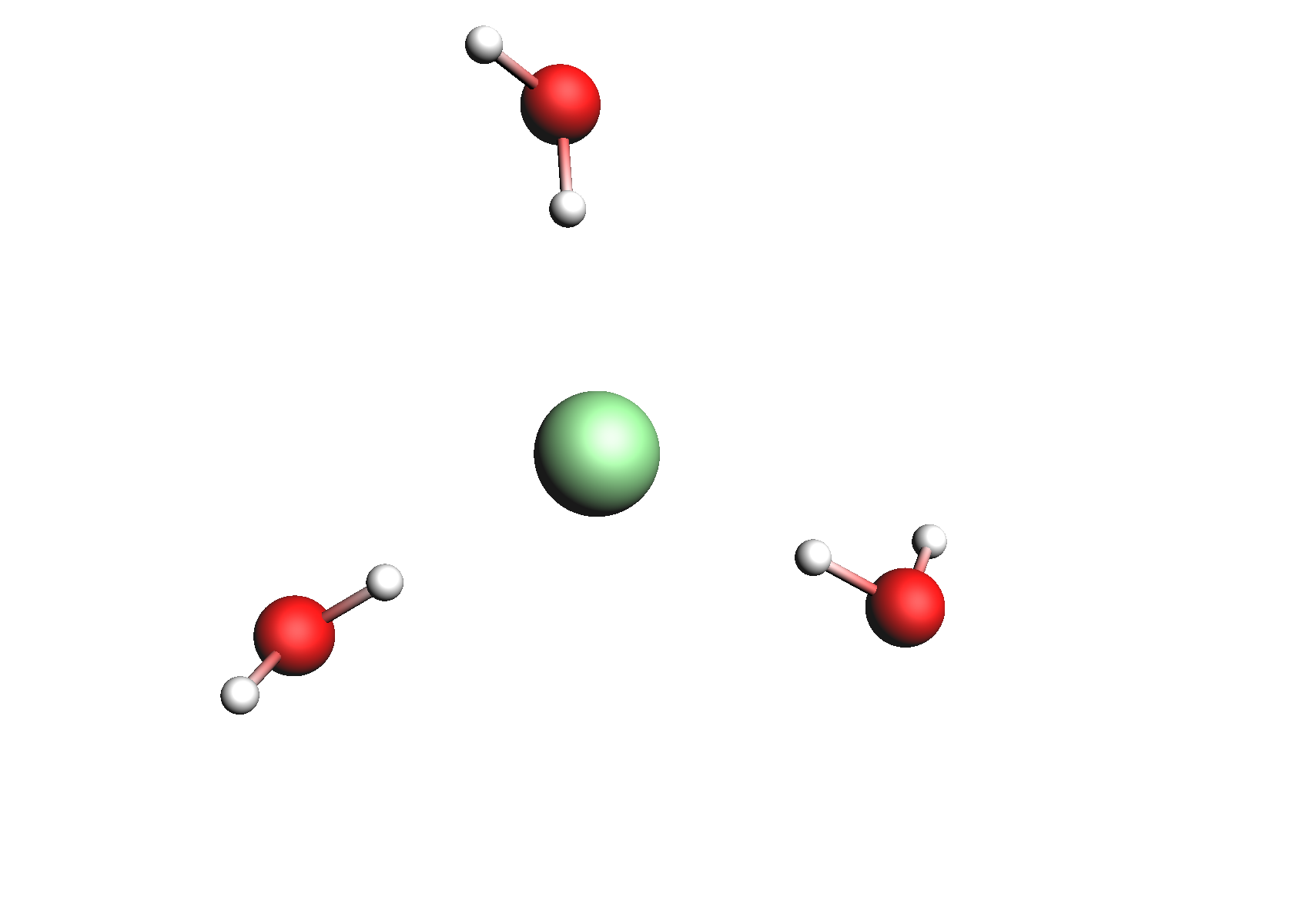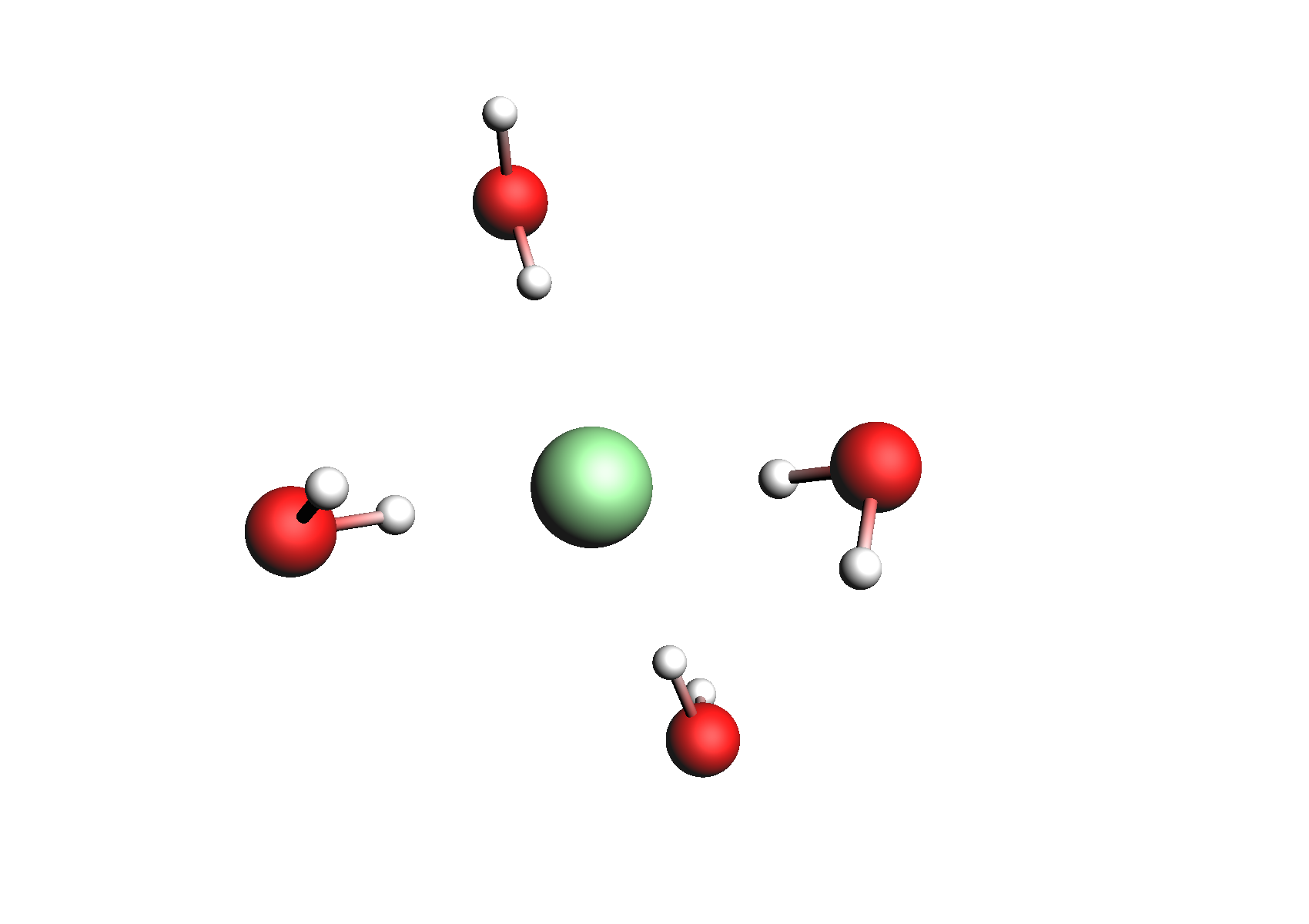Structures used for the Cl- anion (unassociated and 1,2,3,4 explicit waters)

Before we create the multiform files. One important factor to consider is the entropy loss due to the strong association of the water molecules around the ions. Referring to , we use the values -0.021, -0.045, -0.068, -0.093 kcal/(mol K) for the hydration entropies of Na+(H2O), Na+(H2O)2, Na+(H2O)3, Na+(H2O)4, respectively. Similarly, we use the values -0.016, -0.035, -0.054, -0.075 kcal/(mol K) for the hydration entropies of Cl-(H2O), Cl-(H2O)2, Cl-(H2O)3, Cl-(H2O)4, respectively.

First, we input the multiform version of Na+:

Compounds → List of Added Compounds
Add all of the Na+ .coskf files to the GUI with Add
Compounds → Compound with multiple forms
Hit Clear if not empty
In the conformers box, add the free Na+ ion
In the associated section at the bottom, enter the Na+(H2O) .coskf file in the topmost box below Associated
In the box below, enter Water and make sure the requires count is set to 1
Change the Scorr to -0.021
Click the form a1 dropdown box and change to form a2
In the associated section at the bottom, enter the Na+(H2O)2.coskf file in the topmost box below Associated
In the box below, enter Water and make sure the requires count is set to 2
Change the Scorr to -0.045
Repeat this procedure for the coskf files with 3 and 4 explicit waters
Click Save

At this point the CRS GUI should look like the following: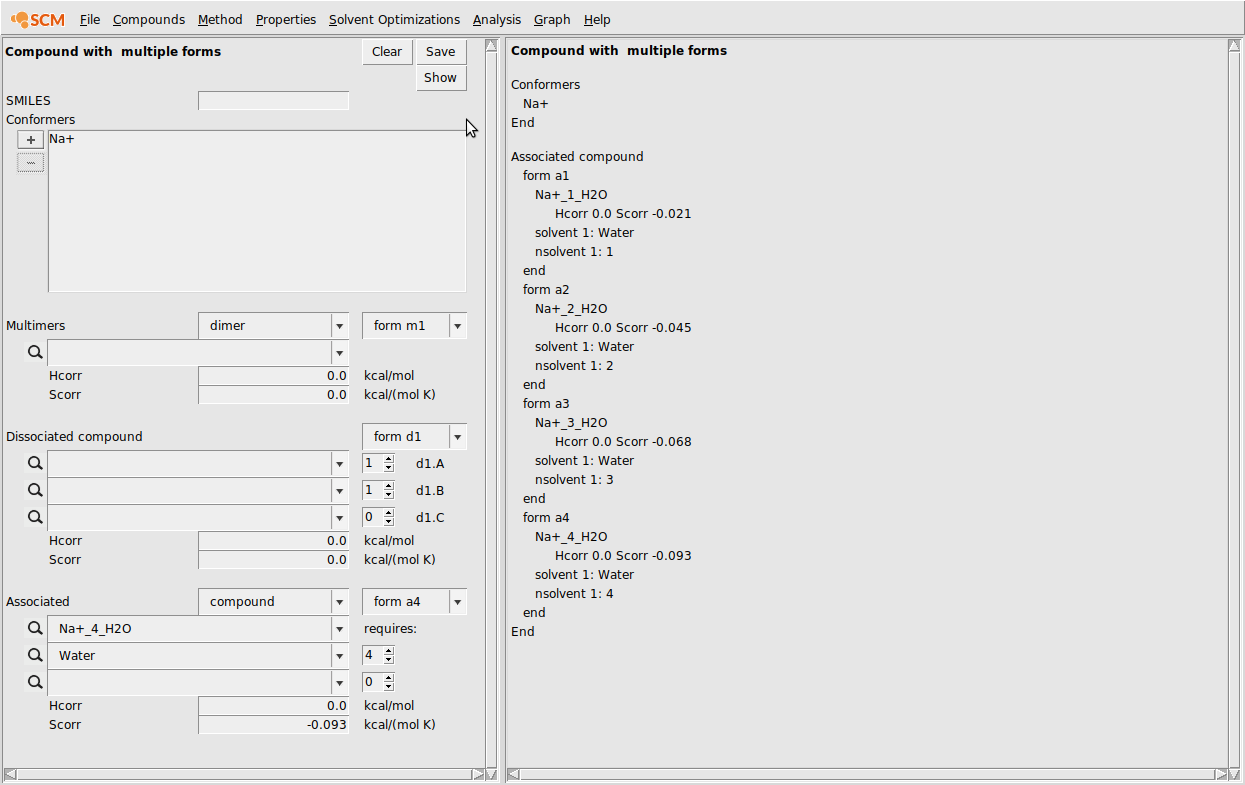Next, we need to input the multiform Cl-:

Compounds → List of Added Compounds
Add all of the Cl- .coskf files to the GUI with Add
Compounds → Compound with multiple forms
Hit Clear if not empty
In the conformers box, add the free Cl- ion
In the associated section at the bottom, enter the Cl-(H2O) .coskf file in the topmost box below Associated
In the box below, enter Water and make sure the requires count is set to 1
Change the Scorr to -0.016
Click the form a1 dropdown box and change to form a2
In the associated section at the bottom, enter the Cl-(H2O)2.coskf file in the topmost box below Associated
In the box below, enter Water and make sure the requires count is set to 2
Change the Scorr to -0.035
Repeat this procedure for the coskf files with 3 and 4 explicit waters
Click Save

The result of this procedure should be the following: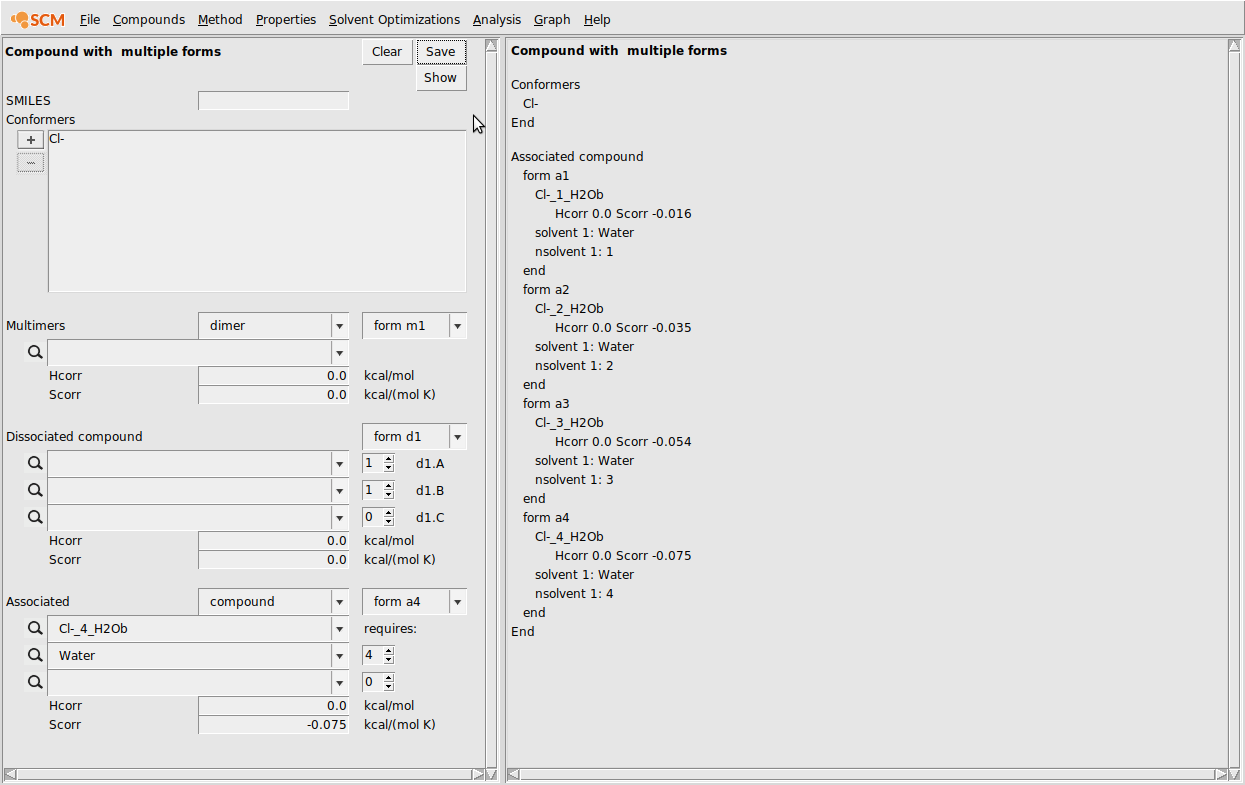Finally, we run calculations with these compounds. We’ll do a solvent composition line calculation:

Properties → Solvents s1-s2 composition line
Choose 3 components
Enter the .multiform file for Na+ in the first box and set (s1,s2) to (0.5,0.0)
Enter the .multiform file for Cl- in the second box and set (s1,s2) to (0.5,0.0)
Enter the .coskf file for Water in the third box and set (s1,s2) to (0.0,1.0)
Change n to 20
Hit Run
Hit Graph → Y axes → total and partial solvent vapor pressure

This should produce the following result: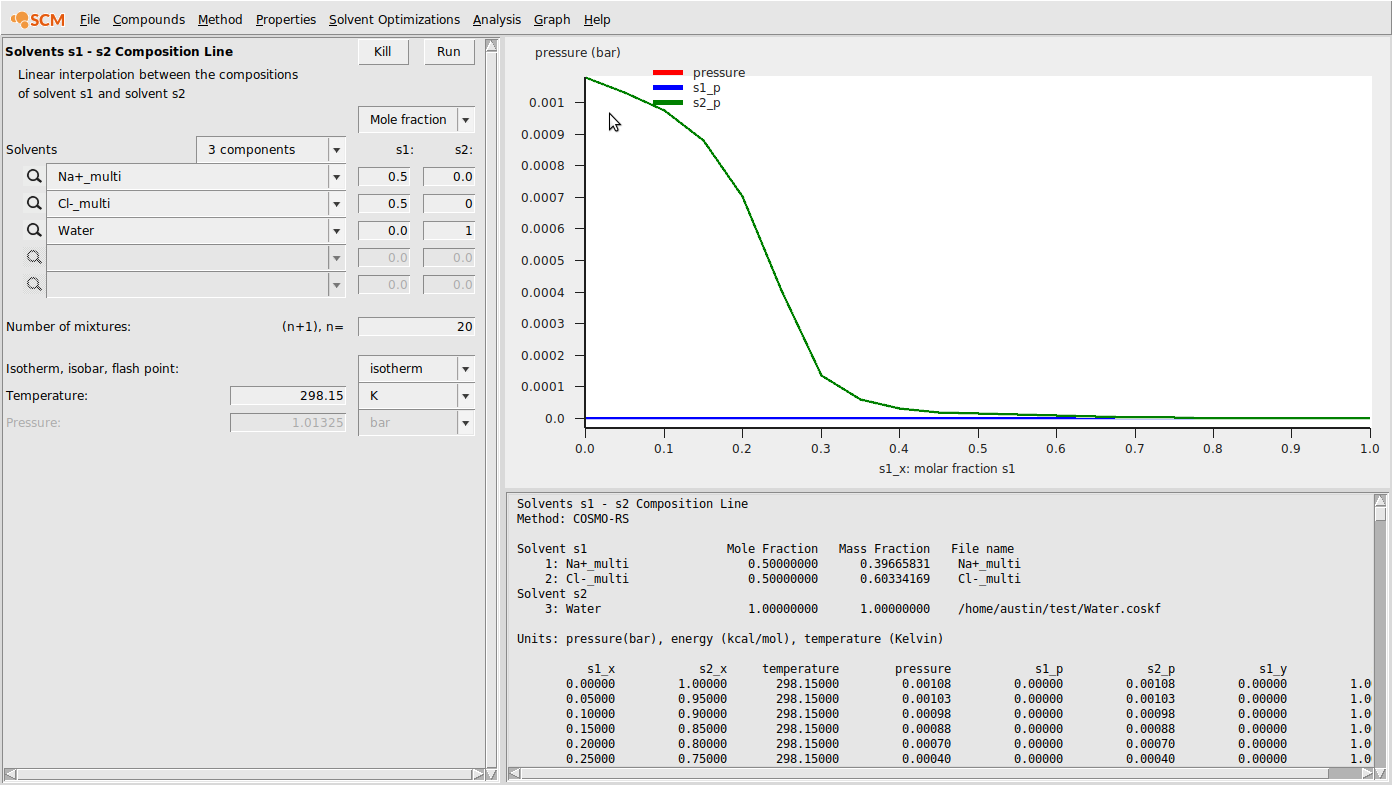As ionic activity coefficients are more commonly reported as mean ionic activity coefficients, we’ll calculate a few of those here. The formula for mean ionic activity coefficients is the following:

$\gamma^±_{NaCl} = \sqrt{ \gamma_{Na+} \gamma_{Cl-} }$

For electrolytes, the reference state is often taken to be at infinite dilution in the solvent. Of course, in the limit of infinite dilution, the chemical potential goes to $$-\infty$$, but we can use the chemical potential calculated with COSMO-RS (the so-called Ben-Naim chemical potential) as it contains no concentration term. We can then calculate the activity coefficients of each species as follows:

$\gamma_i = \exp{ \frac{\mu_i - \mu_i^*}{RT} }$

where $$\mu_i$$ is the chemical potential of an ion calculated by COSMO-RS and $$\mu_i^*$$ is the chemical potential of that ion at infinite dilution. These values can be updated to account for long-range electrostatic effects using the PDHS model described in . Adding that term looks as follows

$\gamma^±_{NaCl} = \gamma^{±,SR}_{NaCl} \gamma^{±,LR}_{NaCl}$

where $$\gamma^{±,SR}_{NaCl}$$ is calculated as shown above and $$\gamma^{±,LR}_{NaCl}$$ comes from the PDHS term. Finally, to compare to experimental data, we’ll have to change to a molality scale for activity. That is done as follows:

$\gamma_i^{(m)} = \frac{ \gamma_i^{(x)}}{1+(\nu^+ + \nu^-) 0.001 M_s m_i }$

where $$\gamma_i^{(m)}$$ is the molality-based (mean ionic) activity coefficient and $$\gamma_i^{(x)}$$ is the mole fraction based activity coefficient. $$(\nu^+ + \nu^-)$$ is the count of the number of ions the salt dissociates into (2 for NaCl), $$M_s$$ is the molar mass of the solvent, and $$m_i$$ is the molality of the salt.

Putting all of this together, we can calculate mean ionic activity coefficients. The results for mean ionic activity coefficient and ln(mean ionic activity coefficient) are shown below. Note that standard COSMO-RS is also compared here as “without association”.Additionally, we can plot the activity coefficient for water as a function of molality: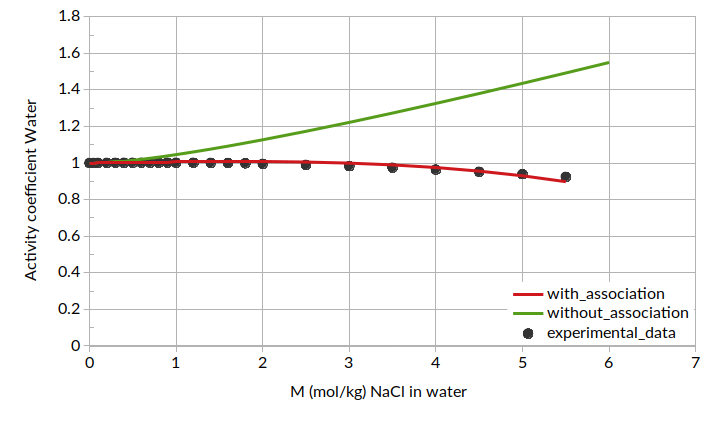References

  Y. Marcus, and A. Loewenschuss, Standard entropies of hydration of ions. Annu. Rep. Prog. Chem., Sect. C: Physical Chemistry 81 (1984) 81-135
  O. Toure, F. Audonnet, A. Lebert, C.-G. Dussap, COSMO-RS-PDHS: A new predictive model for aqueous electrolytes solutions. Chemical Engineering Research and Design 92 (2014) 2873-2883